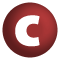# VectorBuilder

#### class VectorBuilder[A] extends Builder[A, Vector[A]] with VectorPointer[A]

attributes: final

### Inherits

1. VectorPointer
2. Builder
3. Growable
4. AnyRef
5. Any

### Value Members

1. #### def ++=(elems: Traversable[A]): Growable[A]

adds all elements contained in a traversable collection to this growable collection

adds all elements contained in a traversable collection to this growable collection.

elems

the collection containing the elements to add.

returns

the growable collection itself.

definition classes: Growable
2. #### def ++=(iter: Iterator[A]): Growable[A]

adds all elements produced by an iterator to this growable collection

adds all elements produced by an iterator to this growable collection.

iter

the iterator producing the elements to add.

returns

the growable collection itself.

definition classes: Growable
3. #### def +=(elem: A): VectorBuilder[A]

Adds a single element to the builder

Adds a single element to the builder.

elem

the element to be added.

returns

the builder itself.

4. #### def +=(elem1: A, elem2: A, elems: A*): Growable[A]

adds two or more elements to this growable collection

adds two or more elements to this growable collection.

elem1

the first element to add.

elem2

the second element to add.

elems

the remaining elements to add.

returns

the growable collection itself

definition classes: Growable
5. #### def clear(): Unit

Clears the contents of this builder

Clears the contents of this builder. After execution of this method the builder will contain no elements.

14. #### def equals(arg0: Any): Boolean

This method is used to compare the receiver object (`this`) with the argument object (`arg0`) for equivalence

This method is used to compare the receiver object (`this`) with the argument object (`arg0`) for equivalence.

The default implementations of this method is an equivalence relation:

• It is reflexive: for any instance `x` of type `Any`, `x.equals(x)` should return `true`.
• It is symmetric: for any instances `x` and `y` of type `Any`, `x.equals(y)` should return `true` if and only if `y.equals(x)` returns `true`.
• It is transitive: for any instances `x`, `y`, and `z` of type `AnyRef` if `x.equals(y)` returns `true` and `y.equals(z)` returns `true`, then `x.equals(z)` should return `true`.

If you override this method, you should verify that your implementation remains an equivalence relation. Additionally, when overriding this method it is often necessary to override `hashCode` to ensure that objects that are "equal" (`o1.equals(o2)` returns `true`) hash to the same `Int` (`o1.hashCode.equals(o2.hashCode)`).

arg0

the object to compare against this object for equality.

returns

`true` if the receiver object is equivalent to the argument; `false` otherwise.

definition classes: AnyRef ⇐ Any
15. #### def hashCode(): Int

Returns a hash code value for the object

Returns a hash code value for the object.

The default hashing algorithm is platform dependent.

Note that it is allowed for two objects to have identical hash codes (`o1.hashCode.equals(o2.hashCode)`) yet not be equal (`o1.equals(o2)` returns `false`). A degenerate implementation could always return `0`. However, it is required that if two objects are equal (`o1.equals(o2)` returns `true`) that they have identical hash codes (`o1.hashCode.equals(o2.hashCode)`). Therefore, when overriding this method, be sure to verify that the behavior is consistent with the `equals` method.

definition classes: AnyRef ⇐ Any
16. #### def mapResult[NewTo](f: (Vector[A]) ⇒ NewTo): Builder[A, NewTo]

Creates a new builder by applying a transformation function to the results of this builder

Creates a new builder by applying a transformation function to the results of this builder.

NewTo

the type of collection returned by `f`.

f

the transformation function.

returns

a new builder which is the same as the current builder except that a transformation function is applied to this builder's result.

definition classes: Builder
17. #### def result(): Vector[A]

Produces a collection from the added elements

Produces a collection from the added elements. The builder's contents are undefined after this operation.

18. #### def sizeHint(size: Int): Unit

Gives a hint how many elements are expected to be added when the next `result` is called

Gives a hint how many elements are expected to be added when the next `result` is called. Some builder classes will optimize their representation based on the hint. However, builder implementations are still required to work correctly even if the hint is wrong, i.e. a different number of elements is added.

definition classes: Builder
19. #### def toString(): String

Returns a string representation of the object

Returns a string representation of the object.

The default representation is platform dependent.

definition classes: AnyRef ⇐ Any